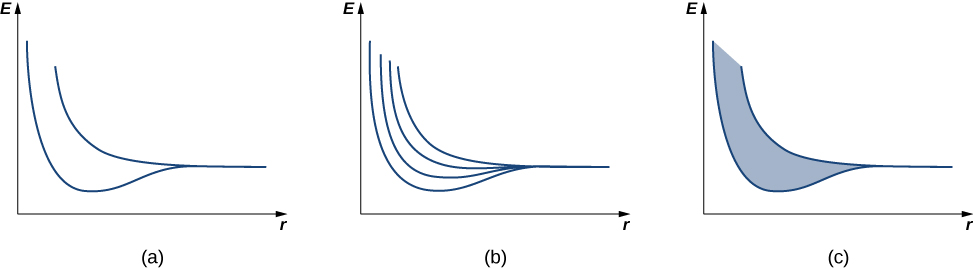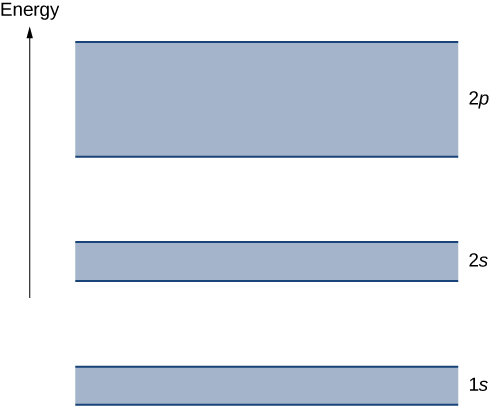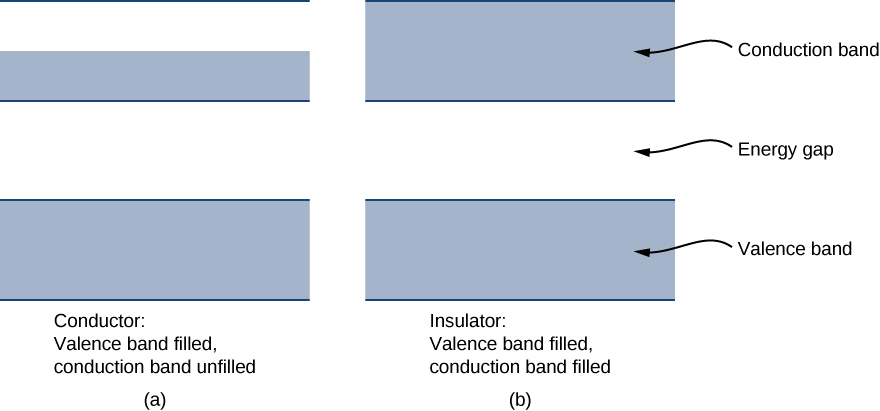# 9.5 Band theory of solids  (Page 2/7)

 Page 2 / 7The dependence of energy-level splitting on the average distance between (a) two atoms, (b) four atoms, and (c) a large number of atoms. For a large number of electrons, a continuous band of energies is produced.A simple representation of the energy structure of a solid. Electrons belong to energy bands separated by energy gaps.

Each energy band is separated from the other by an energy gap    . The electrical properties of conductors and insulators can be understood in terms of energy bands and gaps. The highest energy band that is filled is known as a valence band    . The next available band in the energy structure is known as a conduction band    . In a conductor, the highest energy band that contains electrons is partially filled, whereas in an insulator, the highest energy band containing electrons is completely filled. The difference between a conductor and insulator is illustrated in [link] .

A conductor differs from an insulator in how its electrons respond to an applied electric field. If a significant number of electrons are set into motion by the field, the material is a conductor. In terms of the band model, electrons in the partially filled conduction band gain kinetic energy from the electric field by filling higher energy states in the conduction band. By contrast, in an insulator, electrons belong to completely filled bands. When the field is applied, the electrons cannot make such transitions (acquire kinetic energy from the electric field) due to the exclusion principle. As a result, the material does not conduct electricity.Comparison of a conductor and insulator. The highest energy band is partially filled in a conductor but completely filled in an insulator.

Visit this simulation to learn about the origin of energy bands in crystals of atoms and how the structure of bands determines how a material conducts electricity. Explore how band structure creates a lattice of many wells.

A semiconductor    has a similar energy structure to an insulator except it has a relatively small energy gap between the lowest completely filled band and the next available unfilled band. This type of material forms the basis of modern electronics. At $T=0\phantom{\rule{0.2em}{0ex}}\text{K}$ , the semiconductor and insulator both have completely filled bands. The only difference is in the size of the energy gap (or band gap ) E g between the highest energy band that is filled (the valence band) and the next-higher empty band (the conduction band). In a semiconductor, this gap is small enough that a substantial number of electrons from the valence band are thermally excited into the conduction band at room temperature. These electrons are then in a nearly empty band and can respond to an applied field. As a general rule of thumb, the band gap of a semiconductor is about 1 eV. (See [link] for silicon.) A band gap of greater than approximately 1 eV is considered an insulator. For comparison, the energy gap of diamond (an insulator) is several electron-volts.

Energy gap for various materials at 300 k
Material Energy Gap ${E}_{g}\left(\text{eV}\right)$
$\text{Si}$ $1.14$
$\text{Ge}$ $0.67$
$\text{GaAs}$ $1.43$
$\text{GaP}$ $2.26$
$\text{GaSb}$ $0.69$
$\text{InAs}$ $0.35$
$\text{InP}$ $1.35$
$\text{InSb}$ $0.16$
$\text{C}\phantom{\rule{0.2em}{0ex}}\left(\text{diamond}\right)$ $5.48$

## Summary

• The energy levels of an electron in a crystal can be determined by solving Schrödinger’s equation for a periodic potential and by studying changes to the electron energy structure as atoms are pushed together from a distance.
• The energy structure of a crystal is characterized by continuous energy bands and energy gaps.
• The ability of a solid to conduct electricity relies on the energy structure of the solid.

## Conceptual questions

What are the two main approaches used to determine the energy levels of electrons in a crystal?

(1) Solve Schrödinger’s equation for the allowed states and energies. (2) Determine energy levels for the case of a very large lattice spacing and then determine the energy levels as this spacing is reduced.

Describe two features of energy levels for an electron in a crystal.

How does the number of energy levels in a band correspond to the number, N , of atoms.

For N atoms spaced far apart, there are N different wave functions, all with the same energy (similar to the case of an electron in the double well of ${\text{H}}_{2}\right).$ As the atoms are pushed together, the energies of these N different wave functions are split. By the exclusion principle, each electron must each have a unique set of quantum numbers, so the N atoms bringing N electrons together must have at least N states.

Why are some materials very good conductors and others very poor conductors?

Why are some materials semiconductors?

For a semiconductor, there is a relatively large energy gap between the lowest completely filled band and the next available unfilled band. Typically, a number of electrons traverse the gap and therefore the electrical conductivity is small. The properties of a semiconductor are sensitivity to temperature: As the temperature is increased, thermal excitations promote charge carriers from the valence band across the gap and into the conduction band.

Why does the resistance of a semiconductor decrease as the temperature increases?

## Problems

For a one-dimensional crystal, write the lattice spacing ( a ) in terms of the electron wavelength.

What is the main difference between an insulator and a semiconductor?

For an insulator, the energy gap between the valence band and the conduction band is larger than for a semiconductor.

What is the longest wavelength for a photon that can excite a valence electron into the conduction band across an energy gap of 0.80 eV?

A valence electron in a crystal absorbs a photon of wavelength, $\lambda =0.300\phantom{\rule{0.2em}{0ex}}\text{nm}$ . This is just enough energy to allow the electron to jump from the valence band to the conduction band. What is the size of the energy gap?

4.13 keV

what is bohrs model for hydrogen atom
hi
Tr
Hello
Youte
Hi
Nwangwu-ike
hi
Siddiquee
what is the value of speed of light
1.79×10_¹⁹ km per hour
Swagatika
what r dwarf planet
what is energy
কাজের একক কী
Jasim
কাজের একক কী
Jasim
friction ka direction Kaise pata karte hai
friction is always in the opposite of the direction of moving object
Punia
A twin paradox in the special theory of relativity arises due to.....? a) asymmetric of time only b) symmetric of time only c) only time
b) symmetric of time only
Swagatika
fundamental note of a vibrating string
every matter made up of particles and particles are also subdivided which are themselves subdivided and so on ,and the basic and smallest smallest smallest division is energy which vibrates to become particles and thats why particles have wave nature
Alvin
what are matter waves? Give some examples
according to de Broglie any matter particles by attaining the higher velocity as compared to light'ill show the wave nature and equation of wave will applicable on it but in practical life people see it is impossible however it is practicaly true and possible while looking at the earth matter at far
Manikant
a centeral part of theory of quantum mechanics example:just like a beam of light or a water wave
Swagatika
Mathematical expression of principle of relativity
given that the velocity v of wave depends on the tension f in the spring, it's length 'I' and it's mass 'm'. derive using dimension the equation of the wave
What is the importance of de-broglie's wavelength?
he related wave to matter
Zahid
at subatomic level wave and matter are associated. this refering to mass energy equivalence
Zahid
it is key of quantum
Manikant
how those weight effect a stable motion at equilibrium
how do I differentiate this equation- A sinwt with respect to t
just use the chain rule : let u =wt , the dy/dt = dy/du × du/dt : wA × cos(wt)
Jerry
I see my message got garbled , anyway use the chain rule with u= wt , etc...
Jerry
de broglie wave equation
vy beautiful equation
chandrasekhar
what is electro statics
when you consider systems consisting of fixed charges
Sherly
hi ma new
hello
AmalBy Richley CrapoBy Marion CabalfinBy Donyea SweetsBy OpenStaxBy David CoreyBy OpenStaxBy Yasser IbrahimBy OpenStaxBy David CoreyBy Mistry Bhavesh